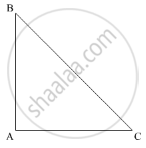Advertisement Remove all ads

# If One Angle of a Triangle is Equal to the Sum of the Other Two Angles, Then the Triangle is - Mathematics

MCQ

If one angle of a triangle is equal to the sum of the other two angles, then the triangle is

#### Options

• an isosceles triangle

• an obtuse triangle

• an equilateral triangle

• a right triangle

Advertisement Remove all ads

#### Solution

In the given problem, one angle of a triangle is equal to the sum of the other two angles.Thus,

∠A = ∠B + ∠C   ..........(1)

Now, according to the angle sum property of the triangle

In ΔABC

∠A + ∠B + ∠C  = 180°

∠B + ∠C +∠B + ∠C  = 180°

2(∠B + ∠C )  = 180°

∠B + ∠C = (180°)/2

∠B + ∠C = 90°                   .........(2)

Further, using (2) in (1),

∠A = ∠B + ∠C

∠A = 90°

Thus, ∠A = 90°

Is there an error in this question or solution?
Advertisement Remove all ads

#### APPEARS IN

RD Sharma Mathematics for Class 9
Chapter 11 Triangle and its Angles
Q 4 | Page 25
Advertisement Remove all ads
Advertisement Remove all ads
Share
Notifications

View all notifications

Forgot password?
Course